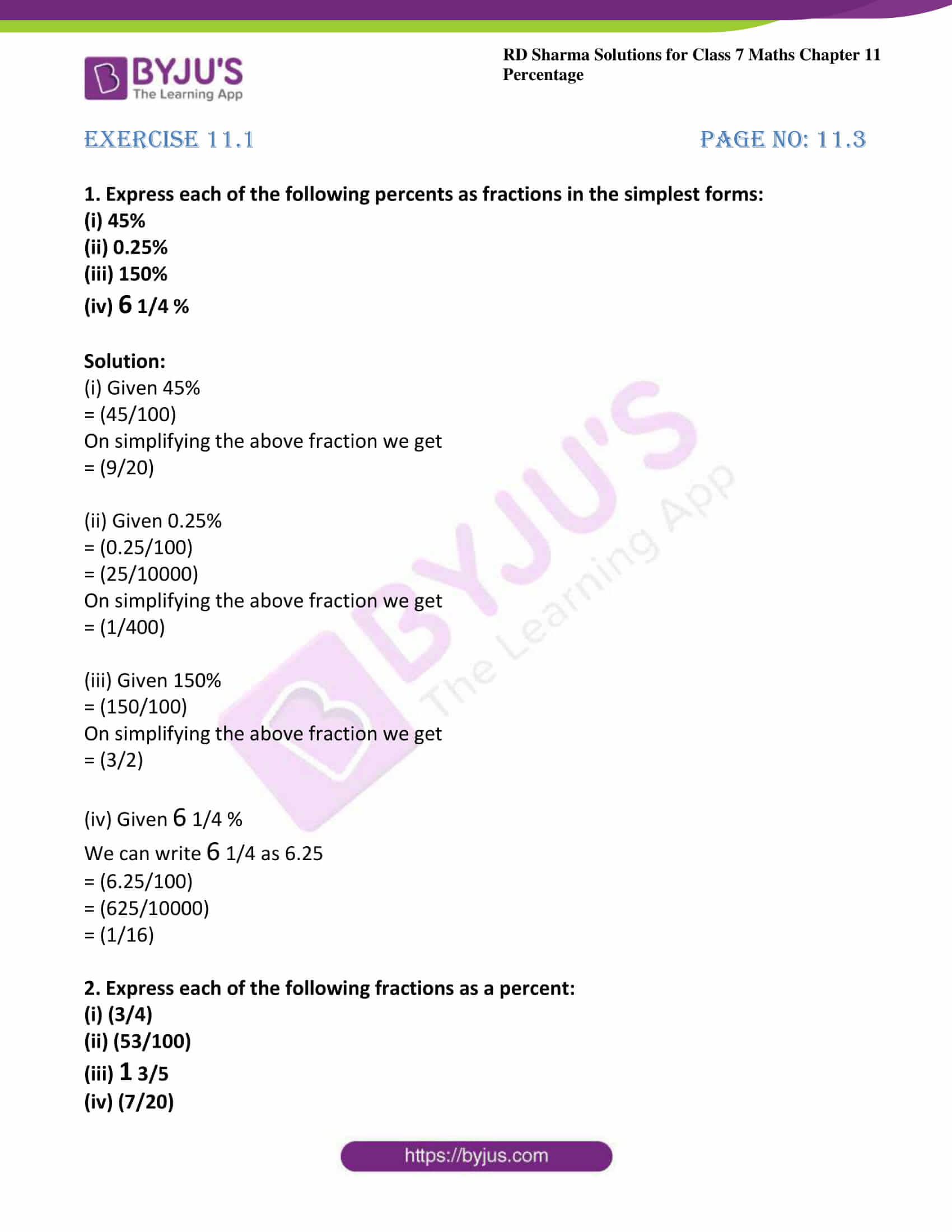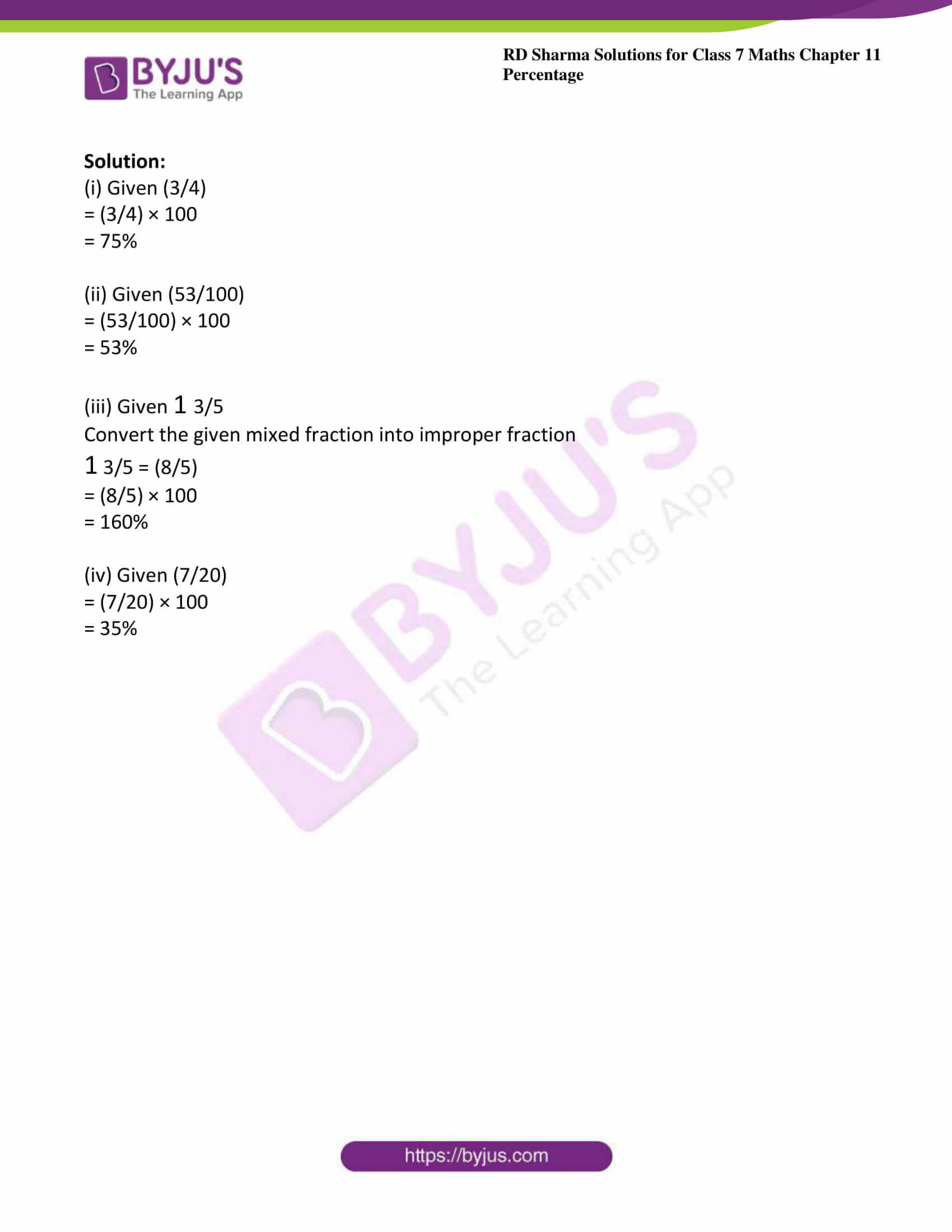# RD Sharma Solutions For Class 7 Maths Chapter 11 Percentage Exercise 11.1

RD Sharma Solutions for Class 7 Maths Exercise 11.1 of Chapter 11 Percentage in PDF are available here. Learners can download the PDF from the provided links. RD Sharma Solutions for Class 7 provides solutions for all topics covered in this exercise. In this exercise, students will get the meaning of percent and the concept of percentage. It mainly deals with conversion of a percent into fraction and conversion of fraction into a percent. Several topics of this exercise are listed below:

• Meaning of percent
• Percent as a fraction
• Percent as a ratio
• Conversion of a percent into a fraction
• Conversion of fraction into percent

## Download the PDF of RD Sharma Solutions For Class 7 Maths Chapter 11 – Percentage Exercise 11.1### Access answers to Maths RD Sharma Solutions For Class 7 Chapter 11 – Percentage Exercise 11.1

Exercise 11.1 Page No: 11.3

1. Express each of the following percents as fractions in the simplest forms:

(i) 45%

(ii) 0.25%

(iii) 150%

(iv) 6 1/4 %

Solution:

(i) Given 45%

= (45/100)

On simplifying the above fraction we get

= (9/20)

(ii) Given 0.25%

= (0.25/100)

= (25/10000)

On simplifying the above fraction we get

= (1/400)

(iii) Given 150%

= (150/100)

On simplifying the above fraction we get

= (3/2)

(iv) Given 6 1/4 %

We can write 6 1/4 as 6.25

= (6.25/100)

= (625/10000)

= (1/16)

2. Express each of the following fractions as a percent:

(i) (3/4)

(ii) (53/100)

(iii) 1 3/5

(iv) (7/20)

Solution:

(i) Given (3/4)

= (3/4) × 100

= 75%

(ii) Given (53/100)

= (53/100) × 100

= 53%

(iii) Given 1 3/5

Convert the given mixed fraction into improper fraction

1 3/5 = (8/5)

= (8/5) × 100

= 160%

(iv) Given (7/20)

= (7/20) × 100

= 35%